# Recall 3D Geometry Notation and Terms

In this worksheet, students will learn the correct terms and notation of 3D shapes.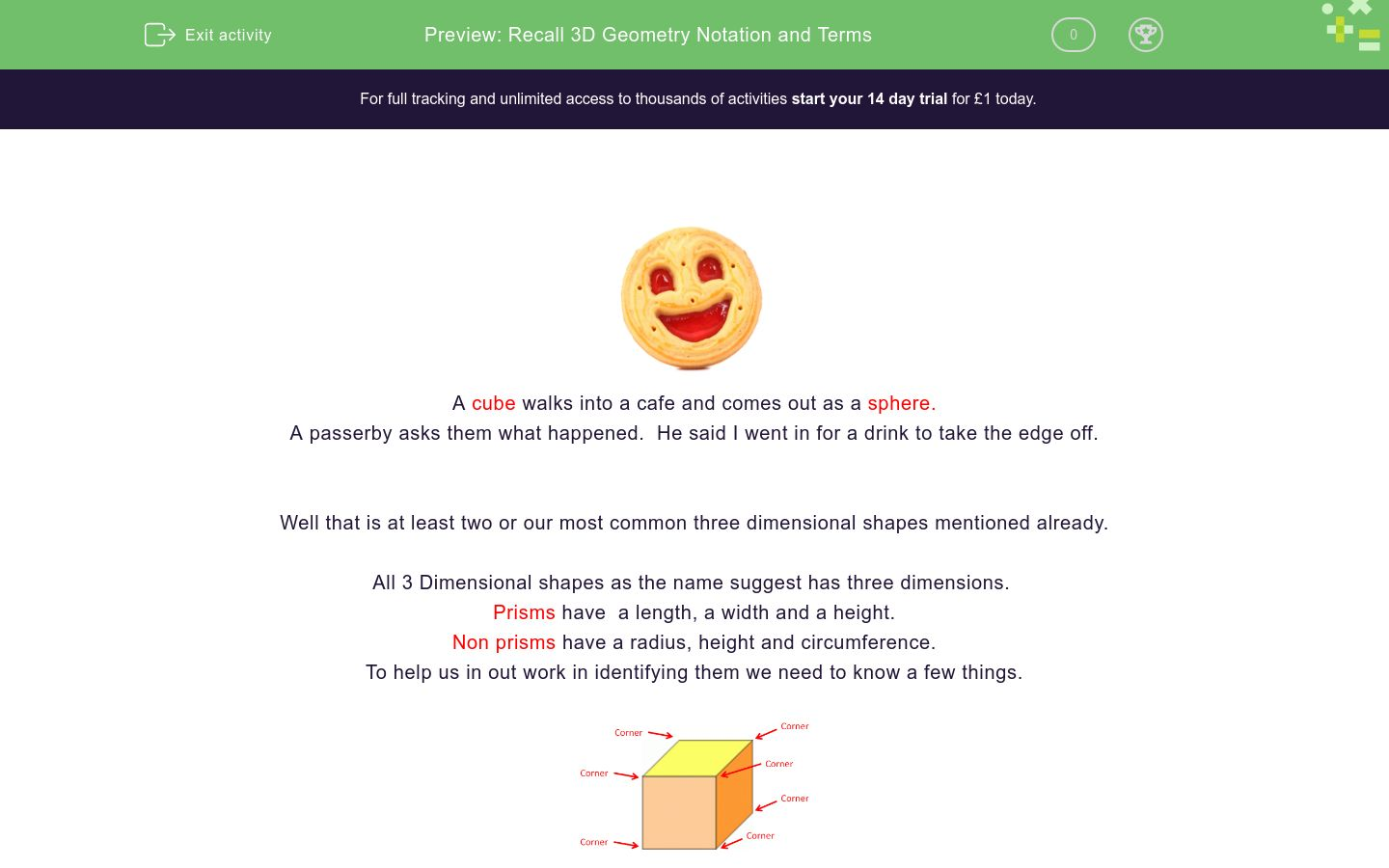Key stage:  KS 4

GCSE Subjects:   Maths

GCSE Boards:   AQA, Eduqas, OCR, Pearson Edexcel

Curriculum topic:   Geometry and Measures, Basic Geometry

Curriculum subtopic:   Properties and Constructions, Conventions, Notations and Terms

Difficulty level:### QUESTION 1 of 10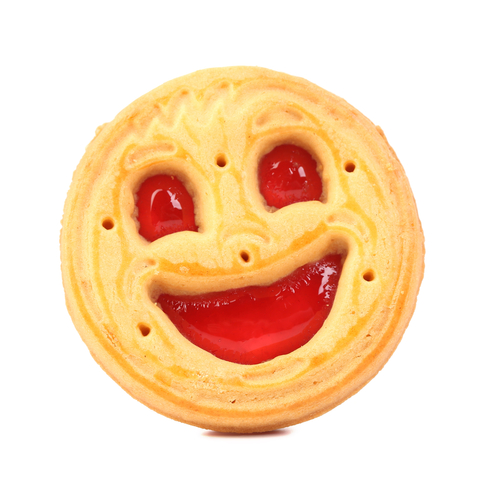A cube walks into a cafe and comes out as a sphere.

A passerby asks them what happened.  He said I went in for a drink to take the edge off.

Well that is at least two or our most common three dimensional shapes mentioned already.

All 3 Dimensional shapes as the name suggest has three dimensions.

Prisms have  a length, a width and a height.

Non prisms have a radius, height and circumference.

To help us in out work in identifying them we need to know a few things.Prisms are identified by the number of vertex, there are. Vertex is the mathematical name for corner.  You need to know this.They are also identified by the number of edges.And finally the number of faces.A sphere is slightly different as it has one smooth surface, with every point on the surface the same distance from the centre.

What are you waiting for.. lets get stuck in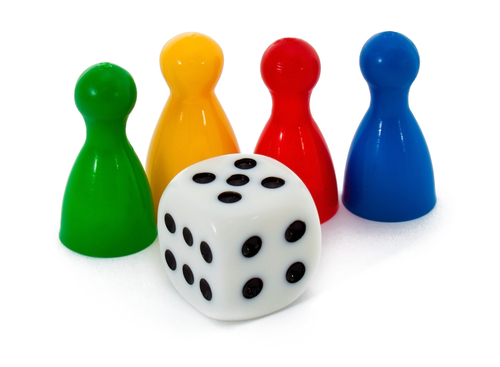How many faces has a cube?A cone A cylinder A triangular pyramid 1 2 3 4 This shape is called The number of faces The number of vertex The number of Edges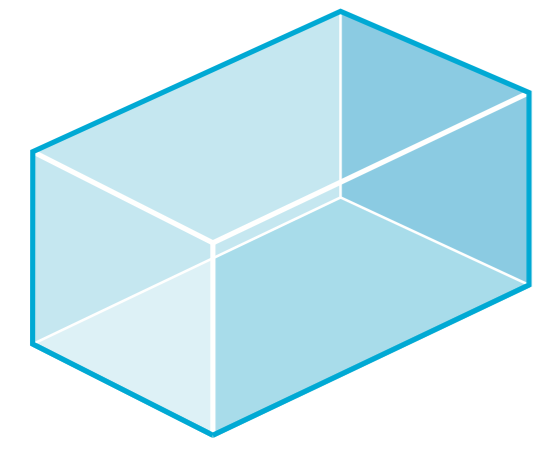This is a cuboid.

 A cone A cylinder A triangular pyramid 1 2 3 4 This shape is called The number of faces The number of vertex The number of Edges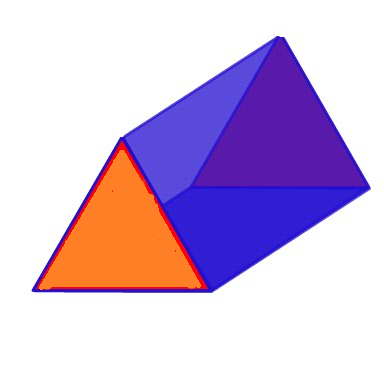Pyramid triangular prism cuboid 4 5 6 7 8 9 What is the name of this shape? How many faces are there? How many edges are there? How many vertices are there?This shape is known as a trapeziodal prism.

 Pyramid triangular prism cuboid 4 5 6 7 8 9 What is the name of this shape? How many faces are there? How many edges are there? How many vertices are there?

Fill in the blanks

 Faces Edges Vertices Cube 8 Square based pyramid 5 Triangular prism 9

 Pyramid triangular prism cuboid 4 5 6 7 8 9 What is the name of this shape? How many faces are there? How many edges are there? How many vertices are there?How many faces does this sphere have?This is a hexagonal prism.

 8 10 12 14 16 18 Number of faces Number of Edges Number of verticesWhat two prisms have the same number of edges, faces and vertices?

Cube

Triangular prism

Trapeziodal prism

Cylinder

Match the description to the shape

## Column B

I have 8 faces and 12 vertices
Pentagonal prism
I have 5 faces and 6 vertices
Triangular prism
I have 3 faces one of them curved
Cylinder
I have no vertices
Hexagonal prism
I have 7 faces and 10 vertices
Sphere
• Question 1How many faces has a cube?

6
EDDIE SAYS
When given a picture of a shape, it is important to count the faces you can't see as well as the ones that you can. I reckon that this dice is being stopped from going into that cafe... a round dice would be no good to anyone.
• Question 2A cone A cylinder A triangular pyramid 1 2 3 4 This shape is called The number of faces The number of vertex The number of Edges
EDDIE SAYS
These can sometimes be tricky because of the curved face. The only edge is the rim on the bottom. The curved part is one of the faces and the base the other.
• Question 3This is a cuboid.

EDDIE SAYS
If you are given a shape with the internal lines shown it is easier to count. If you are shown a shape without, draw them in.
• Question 4Pyramid triangular prism cuboid 4 5 6 7 8 9 What is the name of this shape? How many faces are there? How many edges are there? How many vertices are there?
EDDIE SAYS
The thing most people forget is to count the bottom as one of the faces. I hope you didn't. Have you notice that the edges and vertices are all multiples of three which is the number most associated with the triangle? Full of useful information I am.
• Question 5This shape is known as a trapeziodal prism.

EDDIE SAYS
Are you getting used to counting the faces, edges and vertices? It is useful to tick them off on the shape as you go along so as not to miss anything.
• Question 6

Fill in the blanks

 Faces Edges Vertices Cube 8 Square based pyramid 5 Triangular prism 9

EDDIE SAYS
How did you find this without a diagram? If in doubt sketch them out, it is far easier than trying to do it without.
• Question 7How many faces does this sphere have?

1
EDDIE SAYS
There are no edges or corners to break this up so therefore there is only one face. Why wouldn't the triangle talk to the sphere? Because he thought it was pointless.
• Question 8This is a hexagonal prism.

 8 10 12 14 16 18 Number of faces Number of Edges Number of vertices
EDDIE SAYS
Have you noticed on prisms that the number of faces is 2 more than the number of sides of the shape. i.e 6 sides on a hexagon therefore 8 faces? Have you also noticed that the number of edges and vertices are multiples of the number of sides of the hexagon?
• Question 9What two prisms have the same number of edges, faces and vertices?

Cube
Trapeziodal prism
EDDIE SAYS
The cube and trapezium both have four sides so the rest will be the same. You could of also had a cube and a cuboid, but though that a little to obvious.
• Question 10

Match the description to the shape

## Column B

I have 8 faces and 12 vertices
Hexagonal prism
I have 5 faces and 6 vertices
Triangular prism
I have 3 faces one of them curved
Cylinder
I have no vertices
Sphere
I have 7 faces and 10 vertices
Pentagonal prism
EDDIE SAYS
Did you remember the rule that prisms have two more sides than the shape they are named after and that the vertices are a multiple of the number of sides? This makes it easier than trying to draw the shape out. Happy days..
---- OR ----

Sign up for a £1 trial so you can track and measure your child's progress on this activity.

### What is EdPlace?

We're your National Curriculum aligned online education content provider helping each child succeed in English, maths and science from year 1 to GCSE. With an EdPlace account you’ll be able to track and measure progress, helping each child achieve their best. We build confidence and attainment by personalising each child’s learning at a level that suits them.

Get started